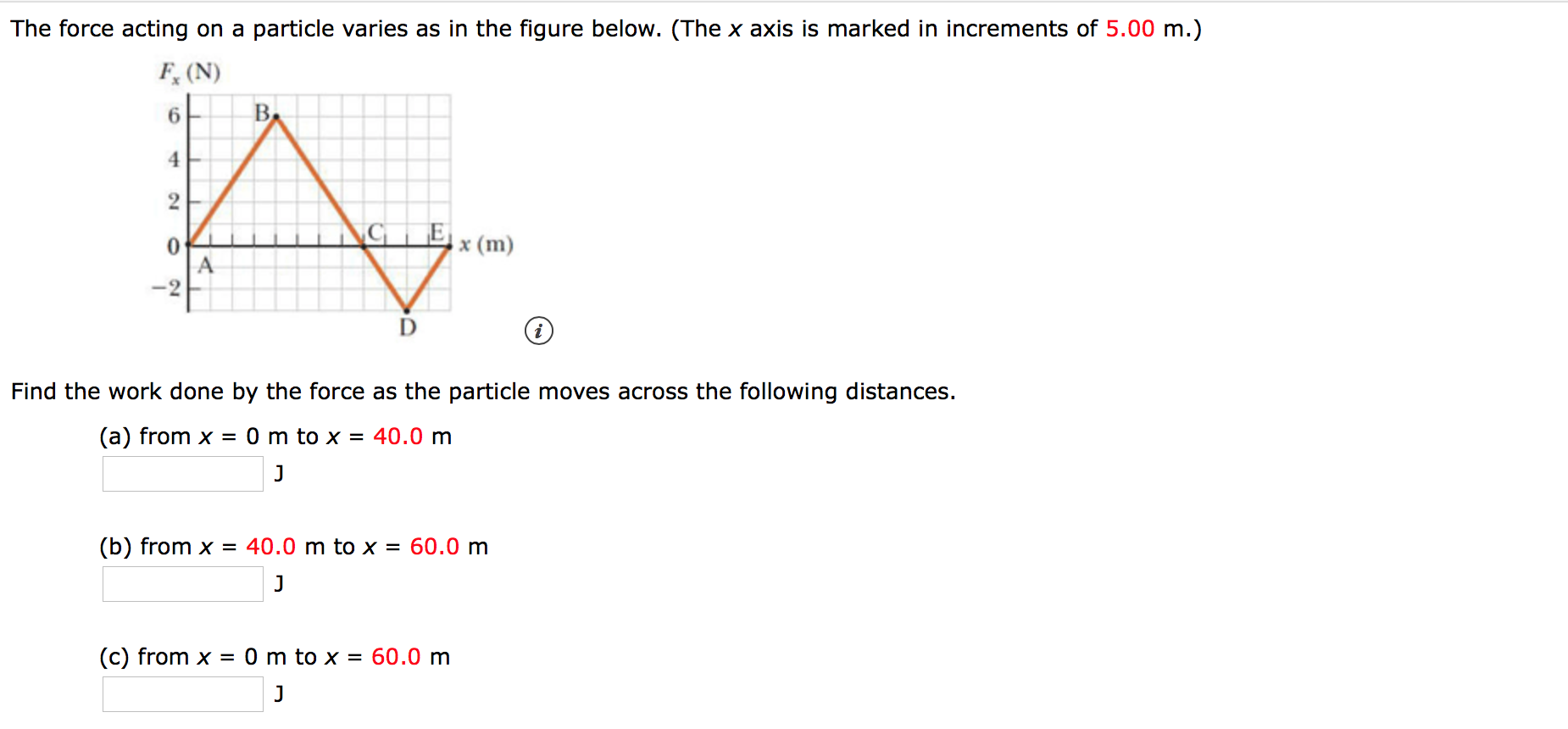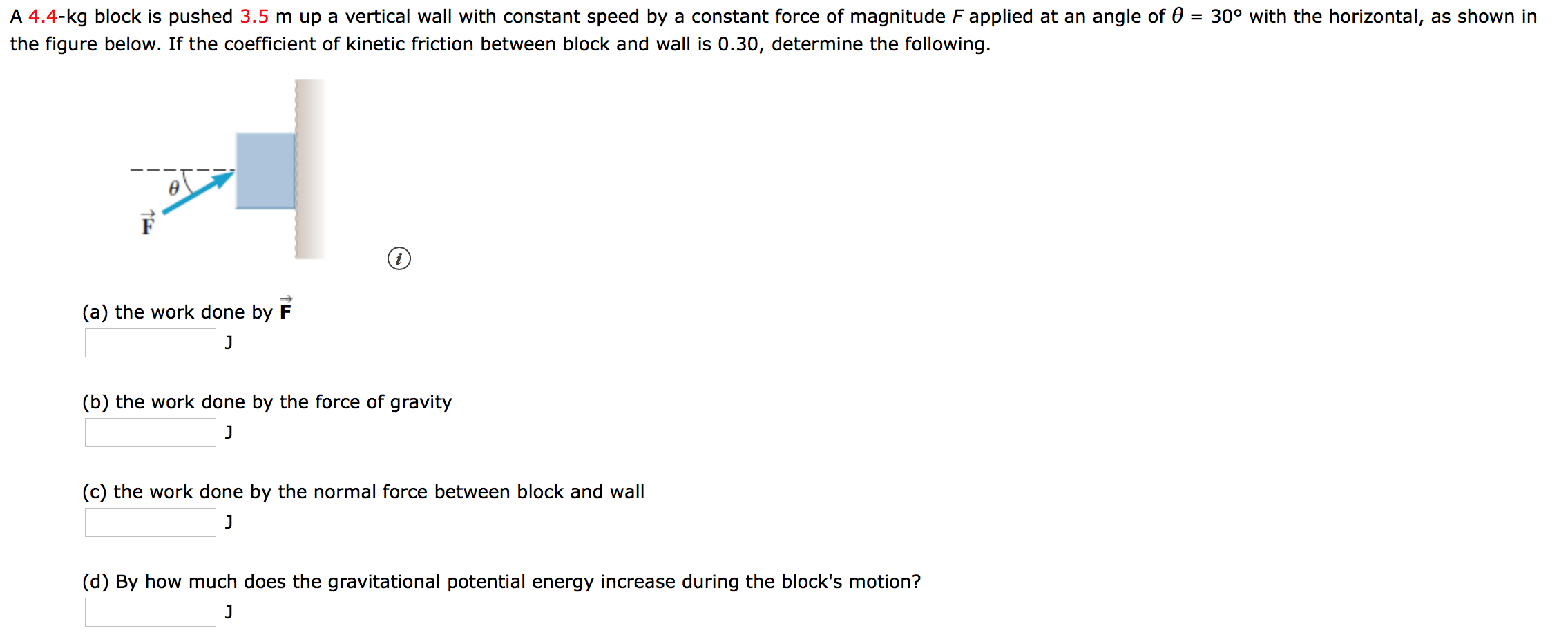# The force acting on a particle varies as in the figure below. (The x axis is marked in increments of 5.00 m.)F(N)В.6E0A-2x (m)VFind the work done by the force as the particle movesthe following distances.(a) from x = 0 m to x = 40.0 mJ40.0 m to x = 60.0 m(b) from xJ(c) from x = 0 m to x = 60.0 mJ A 4.4-kg block is pushed 3.5 m up a vertical wall with constant speed by a constant force of magnitude F applied at an angle of 0 = 30° with the horizontal, as shown inthe figure below. If the coefficient of kinetic friction between block and wall is 0.30, determine the following.(a) the work done by F(b) the work done by the force of gravityJ(c) the work done by the normal force between block and wallJ(d) By how much does the gravitational potential energy increase during the block's motion?J

Question
100 views

i need help with these two questions!help_outlineImage TranscriptioncloseThe force acting on a particle varies as in the figure below. (The x axis is marked in increments of 5.00 m.) F(N) В. 6 E 0 A -2 x (m) V Find the work done by the force as the particle moves the following distances. (a) from x = 0 m to x = 40.0 m J 40.0 m to x = 60.0 m (b) from x J (c) from x = 0 m to x = 60.0 m J fullscreenhelp_outlineImage TranscriptioncloseA 4.4-kg block is pushed 3.5 m up a vertical wall with constant speed by a constant force of magnitude F applied at an angle of 0 = 30° with the horizontal, as shown in the figure below. If the coefficient of kinetic friction between block and wall is 0.30, determine the following. (a) the work done by F (b) the work done by the force of gravity J (c) the work done by the normal force between block and wall J (d) By how much does the gravitational potential energy increase during the block's motion? J fullscreen
check_circle

Step 1

Hey, since there are multiple questions posted, we will answer first question. If you want any specific question to be answered then please submit that question only or specify the question number in your message.

Step 2

(a) The work done by the force as the particle moves from x = 0 m to x = 40.0 m is

Step 3

(b) The work done by the force as the particle...

### Want to see the full answer?

See Solution

#### Want to see this answer and more?

Solutions are written by subject experts who are available 24/7. Questions are typically answered within 1 hour.*

See Solution
*Response times may vary by subject and question.
Tagged in

### Work,Power and Energy